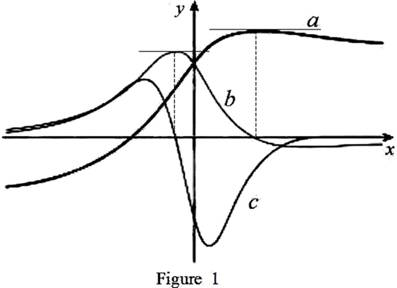# The curves f , f ′ and f ′ ′ on given graph with proper explanation.### Single Variable Calculus: Concepts...

4th Edition
James Stewart
Publisher: Cengage Learning
ISBN: 9781337687805### Single Variable Calculus: Concepts...

4th Edition
James Stewart
Publisher: Cengage Learning
ISBN: 9781337687805

#### Solutions

Chapter 2.7, Problem 41E
To determine

## To identify: The curves f,f′ and f′′ on given graph with proper explanation.

Expert Solution

In the given graph,a=f, b=f and c=f.

### Explanation of Solution

Graph:

The given graph is shown as in Figure 1,Observation:

From the Figure 1, it is observed that the point where c(x)=0 is the same point where graph of b(x) has horizontal tangent.

Recall that the derivative of a function is zero where the function has a horizontal tangent.

Therefore, c(x) is the derivative of the graph b(x). That is, b(x)=c(x).

Observe the graph of a and b carefully.

The point where b(x)=0 is the same point where graph of a(x) has horizontal tangent.

Recall that the derivative of a function is zero where the function has a horizontal tangent.

Therefore, b(x) is the derivative of the graph a(x). That is, a(x)=b(x).

From Figure 1, it is observed that, neither a(x) nor b(x) are equal to zero where the graph of c(x) has horizontal tangent. Clearly, f=c(x).

Use the above information a(x)=b(x) and b(x)=c(x) to concluded that a(x)=c(x).

Thus, a=f,b=f, and c=f.

### Have a homework question?

Subscribe to bartleby learn! Ask subject matter experts 30 homework questions each month. Plus, you’ll have access to millions of step-by-step textbook answers!